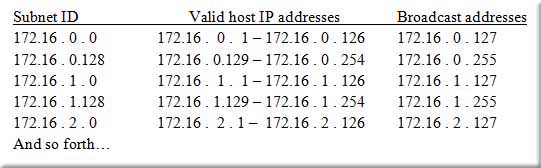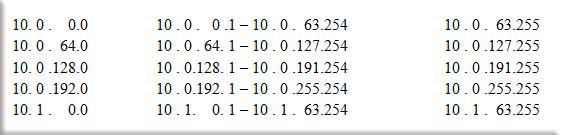# Advanced ‘folding the paper’ subnetting concepts

Home > Blogs > Cisco > Advanced ‘folding the paper’ subnetting concepts

## Advanced ‘folding the paper’ subnetting concepts

3As I mentioned in my previous blog, I want to share with you how to apply the ‘folding the paper’ subnetting method to more advanced subnetting questions. We will examine the concepts of creating more than two subnets and subnetting Class B and Class A networks.

Our first objective is to build on the previous problem where we created only two subnets from one Class C network. This time, let’s start with the same network and mask, which was 192.168.1.0 with a subnet mask of 255.255.255.0. Our task is to create six subnets from this given information by ‘folding’ it. How many times do I have to fold this one network to create at least six subnets? One fold gets me two, two folds gets me four, and three folds gets me eight. Notice I can’t create exactly six equal subnets by folding the original network since I am working with powers of two, and six is not a power of two. So, back to our folds. To achieve the objective, I need to ‘fold’ my network three times. That means I am borrowing three bits from the hosts side and making them network bits. Let’s see how this looks in binary.

New mask with borrowed bits:           11111111.11111111.11111111.11100000

Referencing our chart from the previous blog, and placing the bits:

| 128 |  192| 224 | 240| 248| 252| 254| 255|   <-  Valid subnet mask values

| 128 |  64  |  32  |  16  |  8   |  4    |  2   |   1  |   <-  Decimal weights of the columns

1         1        1        0       0       0        0        0

I have borrowed three bits, which means I have advanced three positions into the chart from the left to the right. This means the value on top in the chart is the new subnet mask value. The new mask in decimal looks like this: 255.255.255.224.
A mask value of 224 gives us what network increment? The number below 224 in the chart is the answer, and that is 32. So our network is incrementing in chunks of 32. In which octet are these increments occurring? Which octet is ‘interesting’? We see that the borrowed bits end (and begin, in this problem) in the fourth octet. This makes that fourth octet ‘interesting.’ We take these two facts and begin to list our information.
Starting position is just the base, or given, network ID: 192 . 168 . 1 . 0

Now write the increment value that was just determined under the beginning network ID in the ‘interesting’ octet position like this:

192 . 168 . 1 . 0

192 . 168 . 1 .32

Once you have done this, it is a straightforward matter to continue to list the network IDs, just using the increment. So add 32 to 32 gives 64, 32 more is 96, and so on. Let’s see how it looks.

192 . 168 . 1 . 0

192 . 168 . 1 .32

192 . 168 . 1. 64

192 . 168 . 1. 96

Now our goal is to determine the directed broadcast addresses, which is the right ‘edge’ of our newly created subnets. I just look at the next starting network ID and subtract one. For example, if the next network starts at .32, then the previous one must end one below that, or .31, which is the directed broadcast addressfor the 192.168.1.0 subnet. Then we just follow that pattern for however many subnets we listed. There is no need to list them all, unless you want to or if the question requires it. So let’s place the directed broadcast addresses for the ones we have listed:

192 . 168 . 1 . 0                      192 . 168 . 1 . 31         (One less than 32)

192 . 168 . 1 .32                      192 . 168 . 1 . 63         (One less than 64)

192 . 168 . 1. 64                      192 . 168 . 1 . 95         (One less than 96)

192 . 168 . 1. 96                      192 . 168 . 1 .127        (One less than 128)

192 . 168 . 1 .128   <– I put this next one to see where it starts so I can stay one below.

I usually list enough subnets so I can see the pattern. If a large number of subnets are created, it serves no purpose to list every single one of them. The first four or five is generally enough to see the pattern.

Now we want to fill in the valid host IP address ranges on the listed subnets. Just add one to the subnet ID for the first one and subtract one from the directed broadcast addressfor the last one. Like this:

192 . 168 . 1 . 0                      192.168.1.1 – 192.168.1.30           192 . 168 . 1 . 31

192 . 168 . 1 .32                      192.168.1.33 – 192.168.1.62              192 . 168 . 1 . 63

192 . 168 . 1. 64                      192.168.1.65 – 192.168.1.94              192 . 168 . 1 . 95

192 . 168 . 1. 96                      192.168.1.97 – 192.168.1.126            192 . 168 . 1 .127

192 . 168 . 1 .128

To review your grasp of the concept, ask yourself: How many networks have I created by borrowing three bits?  Since we know three bits translates to three folds of the network, let’s count by powers of two: |  two, ||  four, |||  eight.  Done!  (The |’s are supposed to be fingers!)  So we have created eight subnets.  This achieves our objective of creating at least six subnets, as posed at the outset.

Now let’s apply this same methodology to a Class B network.  Don’t let that freak you out – the folding doesn’t change a bit.  Let us use, for example, the network 172.16.0.0 with a subnet mask of 255.255.0.0 (which can also be written as 172.16.0.0 /16 using CIDR notation).  We need to create 300 subnets.  Let’s fold it!

Again, since it is a Class B network with the default Class B mask, it is just one network.  How many times must it be folded to get 300 networks?  Imagine raising a finger with every number that follows:   2, 4, 8, 16, 32, 64, 128, 256, 512.  There, we have our first number greater than the number of networks required.  Since nine fingers were raised, nine bits must be borrowed.  The mask, before we subnet it, looks like this:

11111111.11111111.00000000.00000000

If we borrow nine bits, it looks like this:

11111111.11111111.11111111.10000000

Comparing the binary mask with our chart, we find the new decimal mask becomes 255.255.255.128. We see that the borrowed bits span more than one octet. This is no problem; we just have to realize that the number in the third octet will be changing as we roll through the subnet IDs. We will see how that works when we actually list them. Before we do that, let’s determine our increment and interesting octet. The increment, found by looking at the number below the subnet mask value of 128 in our chart, is 128. The interesting octet is the fourth one. But as mentioned, the numbers in the third octet will be changing also. It is helpful to note that if the numbers are changing in more than one octet, the octet(s) other than the far right one will always increment by one. Let’s see that in action. I will list the subnet IDs, the directed broadcast addresses, and the ranges all at once in the following lines.You see the pattern after listing just a few of the networks, broadcast address, and host ranges. You can observe the increment in the fourth octet as it changes by 128 with each subnet. As mentioned, the other octet is incrementing by one. Every two networks, the number goes up by one. Just looking at what is listed, and focusing on the third octet, it goes 0,0, then 1,1, then 2, and follows that pattern until all created subnets are listed. Since borrowing nine bits actually creates 512 subnets, I won’t list them all here.

Let’s try a Class A address. If we start with 10.0.0.0 /8 and are tasked to create 1,000 subnets, can we fold it to achieve that goal? If we raise fingers as we fold, we end up with ten folds, resulting in 1,024 subnets, which meets our goal of 1,000 networks. The mask looked like this before subnetting:

11111111.00000000.00000000.00000000

If we borrow ten bits, it looks like this:

11111111.11111111.11000000.00000000

In decimal (referencing our chart), this becomes 255.255.192.0. Once again, the borrowed bits span more than one octet. The ‘interesting’ octet is the third one. The increment with a .192 mask is 64. Let’s list a few of these:Then the pattern repeats, with the second octet incrementing by one as the subnets fill up.

My next blog will cover subnetting a subnet and using this ‘folding’ idea when asked to create networks for a given number of hosts. Check back in later for more!

Enjoy!
Mark Jacob
Cisco Instructor – Interface Technical Training
Phoenix, AZ

## Videos You May Like

### A Simple Introduction to Cisco CML2

Mark Jacob, Cisco Instructor, presents an introduction to Cisco Modeling Labs 2.0 or CML2.0, an upgrade to Cisco’s VIRL Personal Edition. Mark demonstrates Terminal Emulator access to console, as well as console access from within the CML2.0 product. Hello, I’m Mark Jacob, a Cisco Instructor and Network Instructor at Interface Technical Training. I’ve been using … Continue reading A Simple Introduction to Cisco CML2

### Cable Testers and How to Use them in Network Environments

This content is from our CompTIA Network + Video Certification Training Course. Start training today! In this video, CompTIA Network + instructor Rick Trader demonstrates how to use cable testers in network environments. Let’s look at some tools that we can use to test our different cables in our environment. Cable Testers Properly Wired Connectivity … Continue reading Cable Testers and How to Use them in Network Environments

### OSPF Adjacency Troubleshooting Solution – Getting Close to the OSPF adj

In this video, Cisco CCNA & CCNP instructor Mark Jacob shows how to troubleshoot OSPF Adjacency issues by showing the distance between routers with the show ip ospf neighbor command.